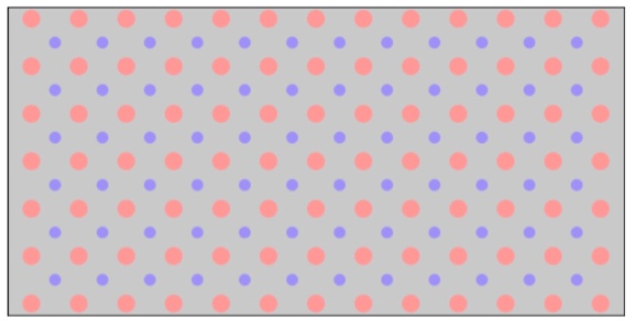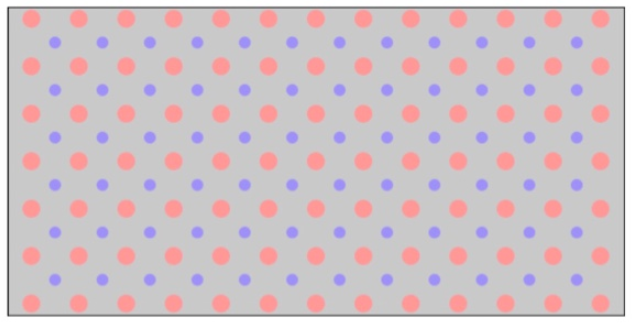# Problem: Drawing the unit cell of a 2D latticeColor in a unit cell of this two-dimensional lattice:

###### FREE Expert Solution

We are asked to color in a unit cell of this two-dimensional lattice:Recall the following definitions:

Lattice points are a periodic array of dots.

Unit cell is the simplest portion of a lattice.

99% (426 ratings)###### Problem Details

Drawing the unit cell of a 2D lattice

Color in a unit cell of this two-dimensional lattice: